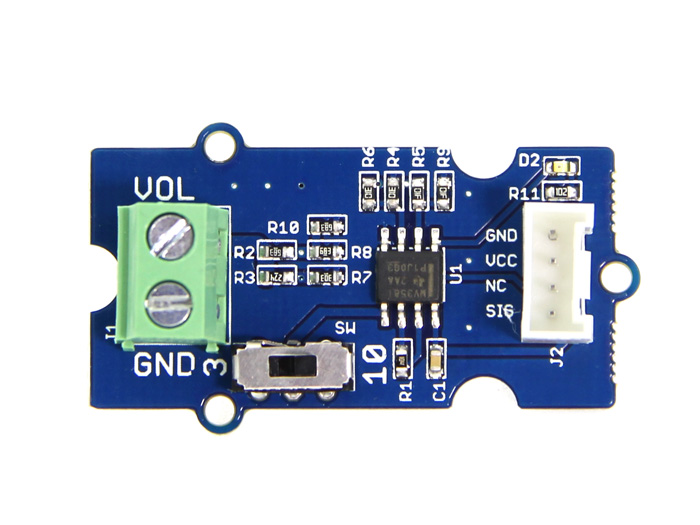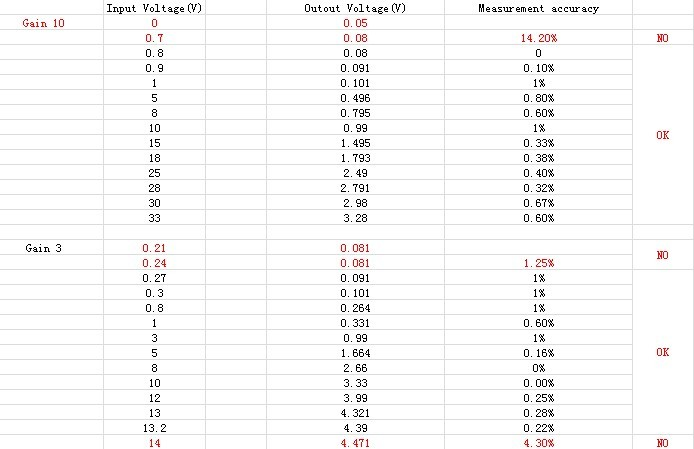<<<<<<< HEAD ======= >>>>>>> 60a8f64d0414dcd5cb8612f64b46979443258e0b Grove-Voltage Divider - Seeed产品文档

# Grove-Voltage DividerGrove – Voltage Divider 提供了测量外部电压的接口，无需将电阻连接到输入接口。另外，电压增益可以通过拨码开关选择，使用方便简单。## 产品特性¶

• 外部电压接口和 Grove 接口
• 使用简便
• 可调整增益

Tip

## 使用方法¶• 正如你所看到的，当输入在测量范围内时，分压器的精确度很高（<1%，我标记为“OK”）。但是，当输入超出范围时，准确度变低（我标记为“ON”）。

• 使用 Arduino 的串行监视器，您可以显示输入电压值。 演示代码如下所示：
void setup()
{
Serial.begin(9600);
}

void loop()
{
long  sum=0;
for(int i=0;i<1000;i++)
{
sum=sensorValue+sum;
delay(2);
}
sum=sum/1000;

Serial.print("if you set the Gain to 10,the input voltage:");
Serial.println(10*sum*4980/1023.00);

Serial.print("if you set the Gain to 3,the input voltage:");
Serial.println(3*sum*4980/1023.00);

delay(1000);
}